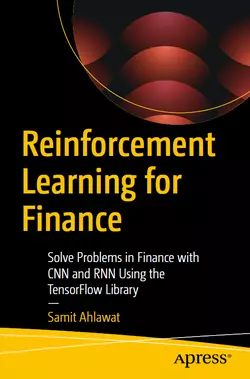# Reinforcement Learning for Finance### eBook Details:

• Paperback: 438 pages
• Publisher: WOW! eBook (December 27, 2022)
• Language: English
• ISBN-10: 1484288343
• ISBN-13: 978-1484288344

### eBook Description:

Reinforcement Learning for Finance: Solve Problems in Finance with CNN and RNN Using the TensorFlow Library

This book introduces reinforcement learning with mathematical theory and practical examples from quantitative finance using the TensorFlow library.

Reinforcement Learning for Finance begins by describing methods for training neural networks. Next, it discusses CNN and RNN – two kinds of neural networks used as deep learning networks in reinforcement learning. Further, the book dives into reinforcement learning theory, explaining the Markov decision process, value function, policy, and policy gradients, with their mathematical formulations and learning algorithms. It covers recent reinforcement learning algorithms from double deep-Q networks to twin-delayed deep deterministic policy gradients and generative adversarial networks with examples using the TensorFlow Python library. It also serves as a quick hands-on guide to TensorFlow programming, covering concepts ranging from variables and graphs to automatic differentiation, layers, models, and loss functions.

• Understand the fundamentals of reinforcement learning
• Apply reinforcement learning programming techniques to solve quantitative-finance problems
• Gain insight into convolutional neural networks and recurrent neural networks
• Understand the Markov decision process

After completing this Reinforcement Learning for Finance book, you will understand reinforcement learning with deep q and generative adversarial networks using the TensorFlow library.